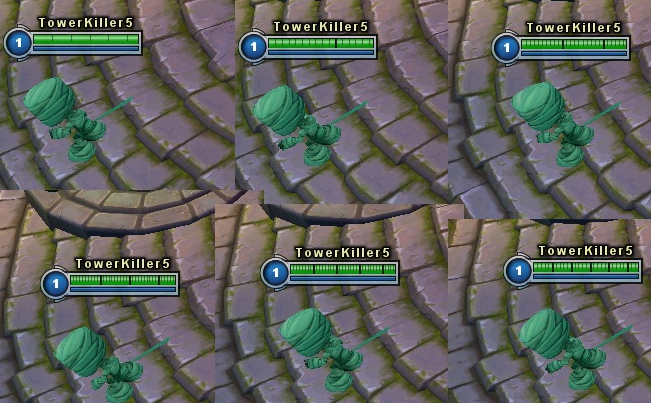# Health Bar Division like in MOBA

I want the health bar in my game to do like in Moba: divide the hp bar in chunks, with a black line. Exemple: You have 200hp? Then you’ll see one line in the middle, 300hp? 2 lines. How do we do this?Well, you already know how many pixels the healthbar is wide, and you also know the HP number. This allows you to calculate how many pixels each HP has:

``````int hpBarWidth = 128;
int hp_max = 214;

float pixelsPerHP = (float)hpBarWidth / hp_max;//~0.6f
int everyHundredHPLineOffset = Mathf.RoundtoInt(pixelsPerHP * 100);//~60
``````

So that means the black line for every 100 HPs will be ~60 pixels offset horizontally from the start of the bar or the last dividing line.

To actually draw the lines, you could use a simple loop that adds 100 the a temporary value equal to the maximum HPs, and add 100 to it for every iteration. The number of the current iteration would then be multiplied with the offset to get the final offset of the current line.

``````int numberOfLines = Mathf.RoundToInt((float)hp_max / 100);
for(int i = 1; i < numberOfLines + 1; i++) {

int offset_current = i * everyHundredHPLineOffset;
//Draw line using offset_current

}
``````